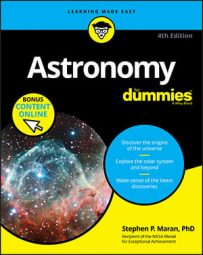##### Astronomy For DummiesEver since the work of Sir Isaac Newton, the English scientist (1642–1727), everything in astronomy has revolved around gravity. Newton explained gravity as a force between any two objects. The force depends on mass and separation. The more massive the object, the more powerful its pull. The greater the distance, the weaker the gravitational attraction. Newton sure was a smart cookie!

Albert Einstein developed an improved theory of gravity, which passes experimental tests that Newton's theory flunks. Newton's theory was good enough for commonly experienced gravity, like the force that made the apple fall on his head (if it really hit him). But in other respects, Newton's theory was hit or miss. Einstein's theory is better because it predicts everything that Newton got right, but also predicts effects that happen close to massive objects, where gravity is very strong. Einstein didn't think of gravity as a force; he considered it the bending of space and time by the very presence of a massive object, such as a star.

Newton's concept of gravity explains the following:

• Why the Moon orbits Earth, why Earth orbits the Sun, why the Sun orbits the center of the Milky Way, and why many other objects orbit one object or another out there in space
• Why a star or a planet is round
• Why gas and dust in space may clump together to form new stars
Einstein's theory of gravity, called the General Theory of Relativity, explains everything that Newton's theory does plus the following:
• Why stars visible near the Sun during a total eclipse seem slightly out of position
• Why black holes exist
• Why gravitational lensing is found when you observe deep space
• Why Earth drags warped space and time around with it as it turns, an effect that scientists have verified with the help of satellites orbiting Earth
• How a collision of two black holes produces gravitational waves that shake things up even billions of light-years away